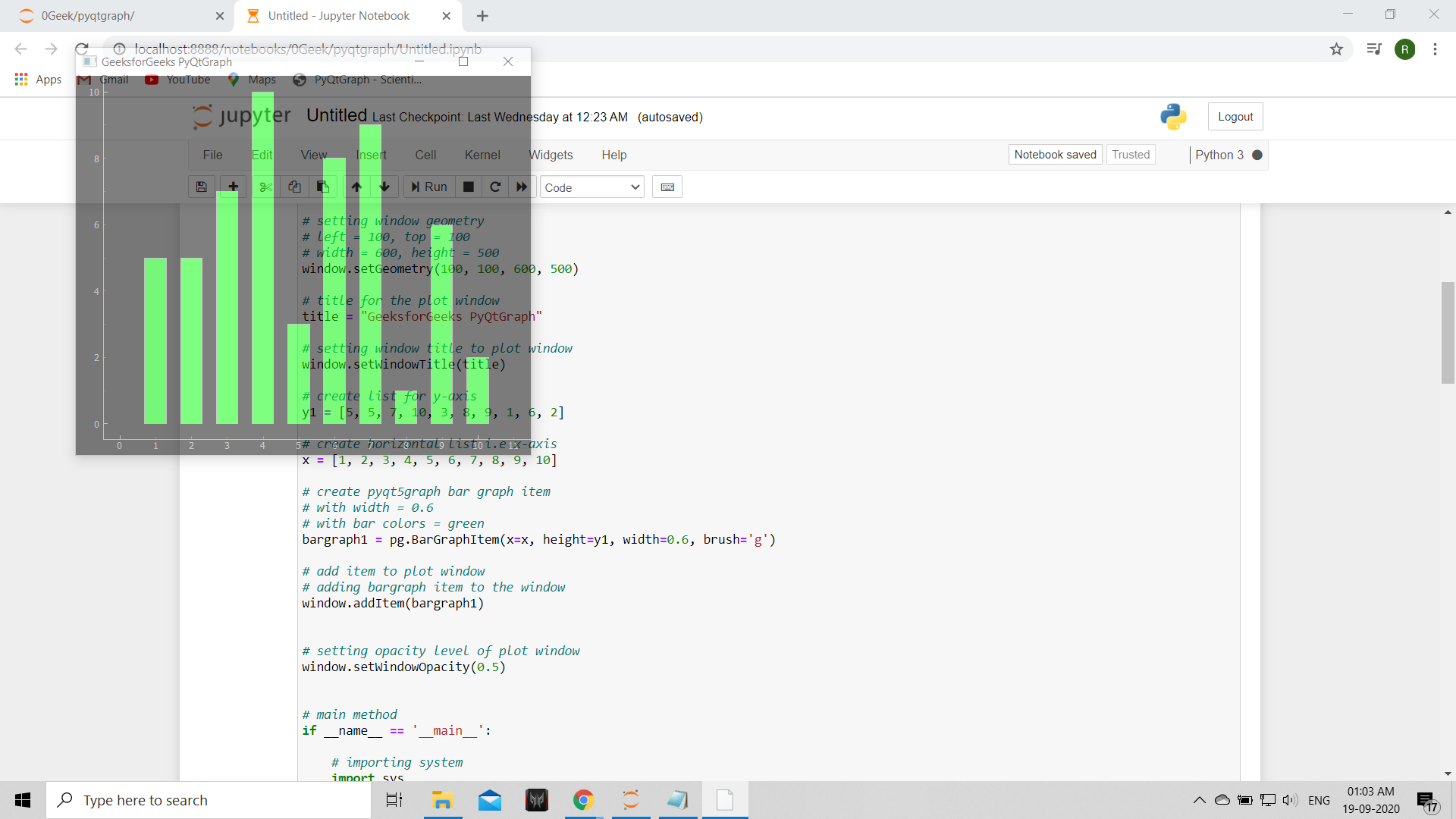# PyQtGraph – Getting Plot Window Opacity

In this article we will see how we can get the opacity of the plot window in the PyQtGraph module. PyQtGraph is a graphics and user interface library for Python that provides functionality commonly required in designing and science applications. Its primary goals are to provide fast, interactive graphics for displaying data (plots, video, etc.) and second is to provide tools to aid in rapid application development (for example, property trees such as used in Qt Designer).Plot windows consist of two main parts: the Plot Panel containing the actual plotted graphics and the Control Panel. Opacity is the measure of impenetrability to electromagnetic or other kinds of radiation, especially visible light. In radiative transfer, it describes the absorption and scattering of radiation in a medium, such as a plasma, dielectric, shielding material, glass, etc. It can be set with the help of `setWindowOpacity` method.

We can create a plot window with the help of command given below

```# creating a pyqtgraph plot window
window = pg.plot()
```

In order to do this we use `windowOpacity` method with the plot window object

Syntax : window.windowOpacity()

Argument : It takes no argument

Return : It returns float value

Below is the implementation

 `# importing pyqtgraph as pg ` `import` `pyqtgraph as pg ` ` `  `# importing QtCore and QtGui from the pyqtgraph module ` `from` `pyqtgraph.Qt ``import` `QtCore, QtGui ` ` `  `# importing numpy as np ` `import` `numpy as np ` ` `  `import` `time ` ` `  `# creating a pyqtgraph plot window ` `window ``=` `pg.plot() ` ` `  `# setting window geometry ` `# left = 100, top = 100 ` `# width = 600, height = 500 ` `window.setGeometry(``100``, ``100``, ``600``, ``500``) ` ` `  `# title for the plot window ` `title ``=` `"GeeksforGeeks PyQtGraph"` ` `  `# setting window title to plot window ` `window.setWindowTitle(title) ` ` `  `# create list for y-axis ` `y1 ``=` `[``5``, ``5``, ``7``, ``10``, ``3``, ``8``, ``9``, ``1``, ``6``, ``2``] ` ` `  `# create horizontal list i.e x-axis ` `x ``=` `[``1``, ``2``, ``3``, ``4``, ``5``, ``6``, ``7``, ``8``, ``9``, ``10``] ` ` `  `# create pyqt5graph bar graph item  ` `# with width = 0.6 ` `# with bar colors = green ` `bargraph1 ``=` `pg.BarGraphItem(x ``=` `x, height ``=` `y1, width ``=` `0.6``, brush ``=``'g'``) ` ` `  `# add item to plot window ` `# adding bargraph item to the window ` `window.addItem(bargraph1) ` ` `  ` `  `# setting opacity level of plot window ` `window.setWindowOpacity(``0.5``) ` ` `  `# getting plot window opacity level ` `value ``=` `window.windowOpacity() ` ` `  `# printing the value ` `print``(``"Window Opacity : "` `+` `str``(value)) ` ` `  ` `  `# main method ` `if` `__name__ ``=``=` `'__main__'``: ` `     `  `    ``# importing system ` `    ``import` `sys ` `     `  `    ``# Start Qt event loop unless running in interactive mode or using  ` `    ``if` `(sys.flags.interactive !``=` `1``) ``or` `not` `hasattr``(QtCore, ``'PYQT_VERSION'``): ` `        ``QtGui.QApplication.instance().exec_() ` `        `

Output :```Window Opacity : 0.4980392156862745
```

Whether you're preparing for your first job interview or aiming to upskill in this ever-evolving tech landscape, GeeksforGeeks Courses are your key to success. We provide top-quality content at affordable prices, all geared towards accelerating your growth in a time-bound manner. Join the millions we've already empowered, and we're here to do the same for you. Don't miss out - check it out now!

Previous
Next